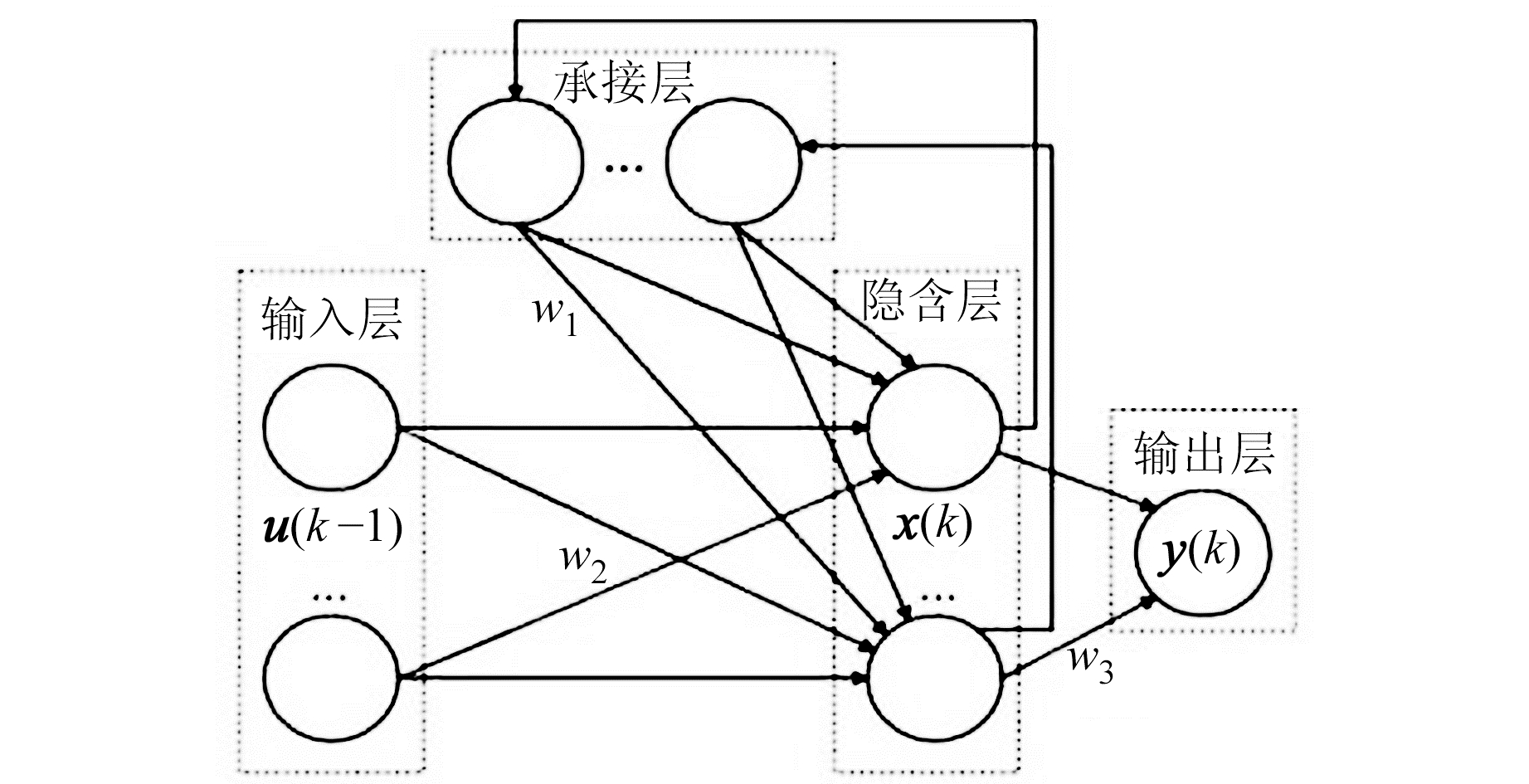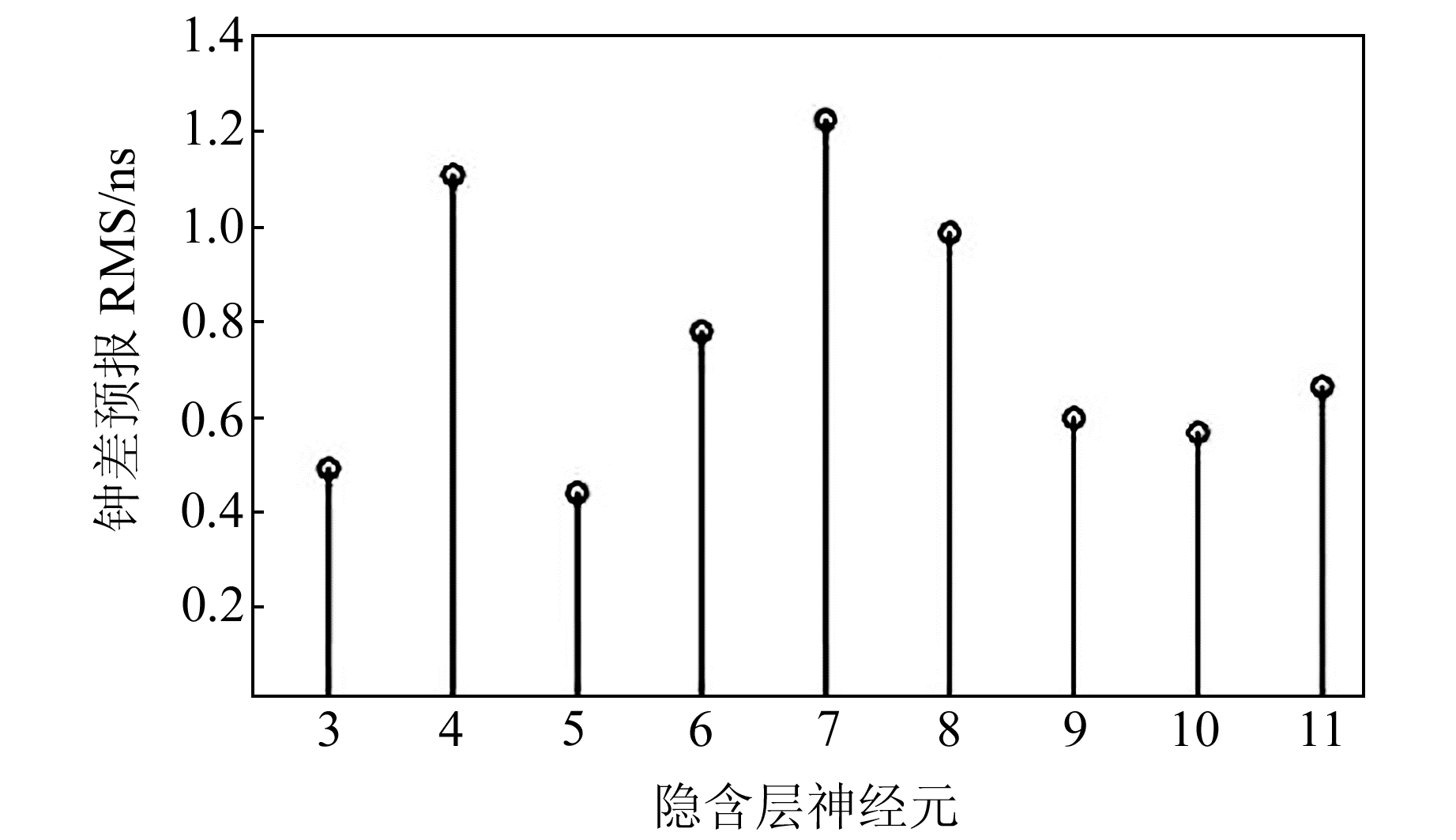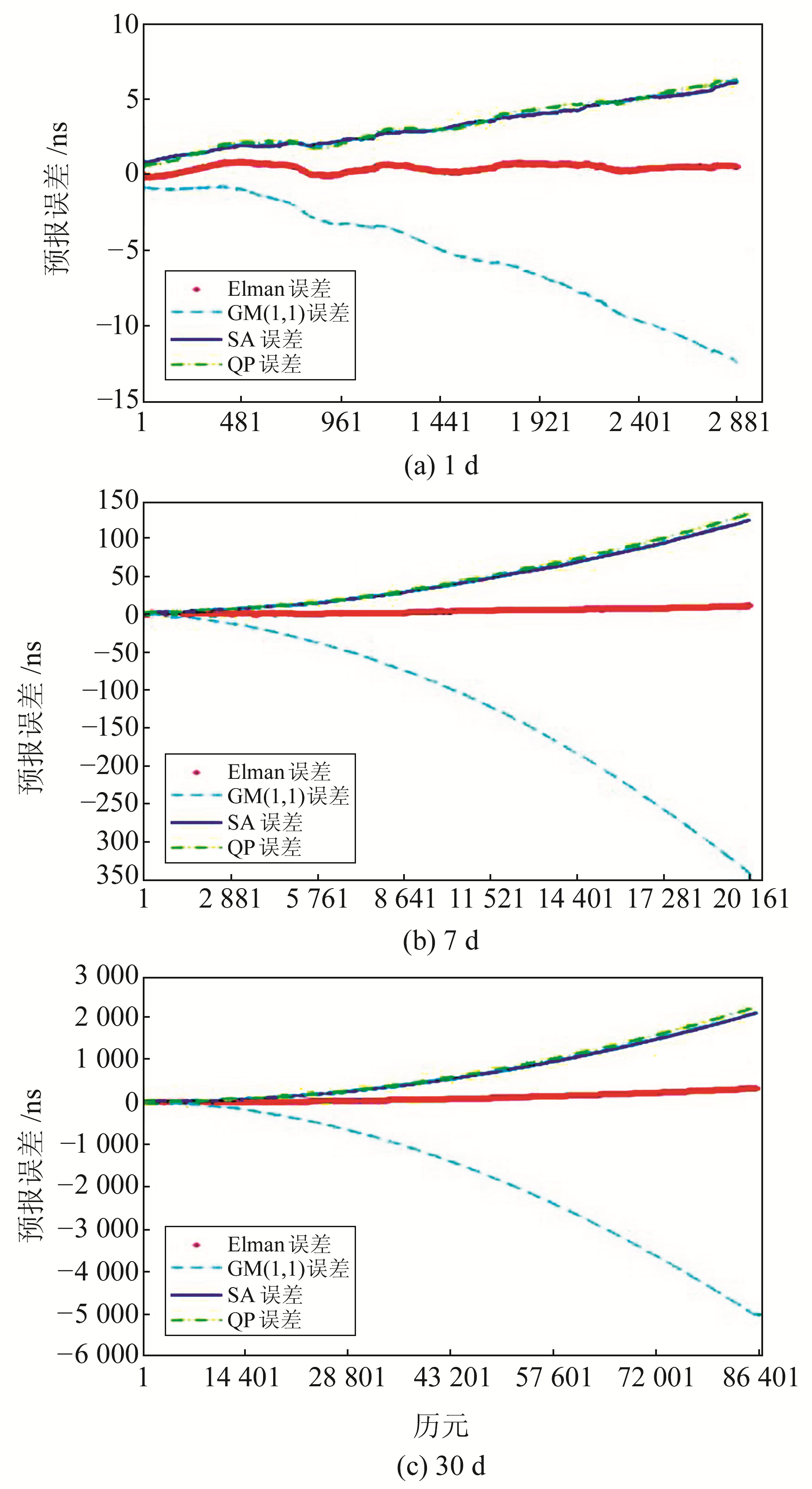﻿ 导航卫星钟差预报的Elman神经网络算法研究文章快速检索 高级检索
 大地测量与地球动力学2021, Vol. 41Issue (3): 285-289, 295  DOI: 10.14075/j.jgg.2021.03.012### 引用本文WANG Run, WANG Jingli, LÜ Dong. Research on the Elman Neural Network Algorithmto Predict Navigation Satellite Clock Bias[J]. Journal of Geodesy and Geodynamics, 2021, 41(3): 285-289, 295.### Corresponding author

WANG Jingli, professor, majors in 3S technology, E-mail: 849552618@qq.com.

### About the first author

WANG Run, postgraduate, majors in prediction algorithm of GNSS satellite clock bias, E-mail: 653440082@qq.com.

### 文章历史

1. 沈阳建筑大学交通工程学院，沈阳市浑南中路25号，110168;
2. 山东科技大学测绘与空间信息学院，青岛市前湾港路579号，266590

1 常用预报模型 1.1 二次多项式模型(QP模型)

 $x_{i}=a_{0}+a_{1}\left(t_{i}-t_{0}\right)+a_{2}\left(t_{i}-t_{0}\right)^{2}+\varepsilon$ (1)

1.2 附加周期项的二次多项式模型(SA模型)

SA模型是在二次多项式模型的基础上附加考虑周期项的钟差预报模型，其表达式为:

 \begin{aligned} x_{i} &=a_{0}+a_{1}\left(t_{i}-t_{0}\right)+a_{2}\left(t_{i}-t_{0}\right)^{2}+\\ & \sum\limits_{k=1}^{p} A_{k} \sin \left[2 \pi f_{k}\left(t_{i}-t_{0}\right)+\varphi_{k}\right]+\varepsilon \end{aligned} (2)

1.3 灰色系统模型(GM(1, 1)模型)

 $x^{(0)}(k+1)=\left(1-\mathrm{e}^{a}\right)\left[x^{(0)}(1)-\frac{u}{a}\right] \mathrm{e}^{-a(k-1)}$ (3)

2 Elman算法基本原理 2.1 Elman神经网络原理

Elman神经网络通过在传统的BP神经网络结构中增加一个承接层，以接收反馈信号和连接记忆上一时刻隐含层的输出状态，并与该时刻的网络输入共同作为隐含层的输入，从而使网络训练具备局部反馈和动态记忆的特性。这种方式使Elman神经网络表现出较强的记忆性和时序性，在关于时间序列的问题上具有较好的适配度。Elman神经网络由输入层、隐含层、承接层和输出层构成，具体如图 1所示。图 1 Elman神经网络结构 Fig. 1 Elman neural network structure

Elman神经网络通过输入层神经元传输信号，同时隐含层神经元采用Sigmoid函数作为激励函数，通过输出层神经元起到线性加权的作用，其表达式为:

 $\begin{array}{c} \boldsymbol{x}(k)=f\left(w_{1} * \boldsymbol{x}_{c}(k)+w_{2} * \boldsymbol{u}(k-1)\right) \end{array}$ (4)
 $\boldsymbol{x}_{c}(k)=\boldsymbol{x}(k-1)$ (5)
 $\boldsymbol{y}_{k}=g\left(w_{3} * \boldsymbol{x}(k)\right)$ (6)

Elman神经网络的连接权值可采用附加动量的梯度下降反向传播算法进行修正，同时将误差平方和函数作为学习指标，其表达式为:

 $E(w)=\sum\limits_{k=1}^{\infty}\left(\boldsymbol{y}_{k}(w)-\tilde{\boldsymbol{y}}_{k}(w)\right)^{2}$ (7)

2.2 Elman神经网络预报GPS卫星钟差

Elman神经网络隐含层神经元个数的确定对模型预报的精度极为关键，如果隐含层的神经元个数较多，则网络的结构会更加稳定，映射非线性函数的能力也将加强，但会降低网络训练的收敛速度; 如果隐含层神经元个数较少，网络结构则会过于简单，计算时间减少，但会导致模型预报能力不足，预报精度也会大大降低。因此需要在保证模型预报能力的前提下，选择合适的隐含层神经元数量。图 2 隐含层神经元变化时钟差预报结果的RMS变化情况 Fig. 2 RMS of clock bias prediction result when hidden layer neuron changes

3 预报实验及分析

 $\mathrm{RMS}=\sqrt{\frac{\sum\limits_{i=1}^{n}\left(\text { error }_{i}\right)^{2}}{n}}$ (8)图 3 钟差预报误差 Fig. 3 Clock bias prediction error表 1 1 d钟差预报结果统计 Tab. 1 1-day clock bias prediction result表 2 7 d钟差预报结果统计 Tab. 2 7-days clock bias prediction result表 3 30 d钟差预报结果统计 Tab. 3 30-days clock bias prediction result

4 结语

1) 在卫星钟差短期预报中，无论是星载铯钟还是星载铷钟，4种模型的钟差预报精度均能达到ns级，而使用Elman模型预报钟差时，部分卫星的预报精度甚至可达到亚ns级，其钟差预报稳定性也有较大提升。

2) 随着预报时长的增加，各类模型进行卫星钟差中长期预报的误差不断累积，导致各模型预报钟差的精度和稳定性迅速降低。但与3种常用模型相比，Elman模型的预报精度和稳定性仍有明显提升。

  王利, 张勤, 黄观文, 等. 基于指数平滑法的GPS卫星钟差预报[J]. 武汉大学学报: 信息科学版, 2017, 42(7): 995-1 001 (Wang Li, Zhang Qin, Huang Guanwen, et al. GPS Satellite Clock Bias Prediction Based on Exponential Smoothing Method[J]. Geomatics and Information Science of Wuhan University, 2017, 42(7): 995-1 001) (0)  郑作亚, 党亚民, 卢秀山, 等. 附有周期项的预报模型及其在GPS卫星钟差预报中的应用研究[J]. 天文学报, 2010, 51(1): 95-102 (Zheng Zuoya, Dang Yamin, Lu Xiushan, et al. Prediction Model with Periodic Item and Its Application to the Prediction of GPS Satellite Clock Bias[J]. Acta Astronomica Sinica, 2010, 51(1): 95-102) (0)  杨宇飞, 杨元喜, 王威, 等. MGEX钟差产品精度分析[J]. 测绘科学技术学报, 2018, 35(5): 441-445 (Yang Yufei, Yang Yuanxi, Wang Wei, et al. Accuracy Assessment for IGS MGEX Clock Products[J]. Journal of Geomatics Science and Technology, 2018, 35(5): 441-445) (0)  Bhattarai S. Satellite Clock Time Offset Prediction in Global Navigation Satellite System[D]. London: University of London, 2015 (0)  Strandjord K L, Axelrad P. Improved Prediction of GPS Satellite Clock Sub-Daily Variations Based on Daily Repeat[J]. GPS Solutions, 2018, 22(3): 58 DOI:10.1007/s10291-018-0723-0 (0)  王宁. BDS星载原子钟钟差数据预处理与钟性能分析研究[D]. 郑州: 信息工程大学, 2017 (Wang Ning. Study on the Methods of Clock Bias Data Preprocessing and Performance Analysis for On-Board Atomic Clocks of BDS[D]. Zhengzhou: Information Engineering University, 2017) (0)  Allan D W. Time and Frequency(Time-Domain) Characterization, Estimation, and Prediction of Precision Clocks and Oscillators[J]. IEEE Transactions on Ultrasonics, Ferroelectrics and Frequency Control, 1987, 34(6): 647-654 DOI:10.1109/T-UFFC.1987.26997 (0)  廖汐琳, 李浩军, 赵润根. GPS卫星钟差高精度模型化及在精密单点定位中的应用[J]. 大地测量与地球动力学, 2018, 38(8): 836-839 (Liao Xilin, Li Haojun, Zhao Rungen. Modeling of GPS Satellite Clock Error and Its Application in Precise Point Positioning[J]. Journal of Geodesy and Geodynamics, 2018, 38(8): 836-839) (0)  李成龙, 陈西宏, 刘继业, 等. 利用自适应TS-IPSO优化的灰色系统预报卫星钟差[J]. 武汉大学学报: 信息科学版, 2018, 43(6): 854-859 (Li Chenglong, Chen Xihong, Liu Jiye, et al. Predicting Satellite Clock Errors Using Grey Model Optimized by Adaptive TS-IPSO[J]. Geomatics and Information Science of Wuhan University, 2018, 43(6): 854-859) (0)  王国成, 柳林涛, 徐爱功, 等. 径向基函数神经网络在GPS卫星钟差预报中的应用[J]. 测绘学报, 2014, 43(8): 803-807 (Wang Guocheng, Liu Lintao, Xu Aigong, et al. The Application of Radial Basis Function Neural Network in the GPS Satellite Clock Bias Prediction[J]. Acta Geodaetica et Cartographica Sinica, 2014, 43(8): 803-807) (0)  Han T. Fractal Behavior of BDS-2 Satellite Clock Offsets and Its Application to Real-Time Clock Offsets Prediction[J]. GPS Solutions, 2020, 24(2): 35 DOI:10.1007/s10291-019-0950-z (0)  马卓希, 杨力, 贾小林. BDS星载原子钟周期项特性及预报研究[J]. 大地测量与地球动力学, 2017, 37(3): 292-296 (Ma Zhuoxi, Yang Li, Jia Xiaolin. Research on Prediction and Characterization of Periodic Variations in BDS Satellite Clocks[J]. Journal of Geodesy and Geodynamics, 2017, 37(3): 292-296) (0)  路晓峰, 杨志强, 贾小林, 等. 灰色系统理论的优化方法及其在卫星钟差预报中的应用[J]. 武汉大学学报: 信息科学版, 2008, 33(5): 492-495 (Lu Xiaofeng, Yang Zhiqiang, Jia Xiaolin, et al. Parameter Optimization Method of Gray System Theory for the Satellite Clock Error Predicting[J]. Geomatics and Information Science of Wuhan University, 2008, 33(5): 492-495) (0)  雷雨, 赵丹宁, 蔡宏兵. 利用结构自适应极端学习机预报导航卫星钟差[J]. 武汉大学学报: 信息科学版, 2018, 43(5): 664-668 (Lei Yu, Zhao Danning, Cai Hongbing. Prediction of Navigation Satellite Clock Offset by Adaptive Extreme Learning Machine[J]. Geomatics and Information Science of Wuhan University, 2018, 43(5): 664-668) (0)  邹丽萍, 宫响, 庄述鹏. 基于Elman神经网络的日总辐射曝辐量预估[J]. 青岛科技大学学报: 自然科学版, 2019, 40(5): 112-118 (Zou Liping, Gong Xiang, Zhuang Shupeng. Estimate of Daily Irradiation Exposure of Global Radiation Using Elman Neural Network[J]. Journal of Qingdao University of Science and Technology: Natural Science, 2019, 40(5): 112-118) (0)  王述红, 任艺鹏, 邢观华. 一种改进AFSA-Elman边坡位移预测网络[J]. 东北大学学报: 自然科学版, 2019, 40(1): 115-120 (Wang Shuhong, Ren Yipeng, Xing Guanhua. An Improved AFSA-Elman Slope Displacement Prediction Network[J]. Journal of Northeastern University: Natural Science, 2019, 40(1): 115-120) (0)  戴文战, 娄海川, 杨爱萍. 非线性系统神经网络预测控制研究进展[J]. 控制理论与应用, 2009, 26(5): 521-530 (Dai Wenzhan, Lou Haichuan, Yang Aiping. An Overview of Neural Network Predictive Control for Nonlinear Systems[J]. Control Theory and Applications, 2009, 26(5): 521-530) (0)  王宇谱, 吕志平, 崔阳, 等. 利用遗传小波神经网络预报导航卫星钟差[J]. 武汉大学学报: 信息科学版, 2014, 39(7): 809-814 (Wang Yupu, Lü Zhiping, Cui Yang, et al. Predicting Navigation Satellite Clock Bias Using a Genetic Wavelet Neural Network[J]. Geomatics and Information Science of Wuhan University, 2014, 39(7): 809-814) (0)  张雨浓, 肖秀春, 陈扬文, 等. Hermite前向神经网络隐节点数目自动确定[J]. 浙江大学学报: 工学版, 2010, 44(2): 271-275 (Zhang Yunong, Xiao Xiuchun, Chen Yangwen, et al. Number Determination of Hidden-Layer Nodes for Hermite Feed-Forward Neural Network[J]. Journal of Zhejiang University: Engineering Science, 2010, 44(2): 271-275) (0)  王宇谱, 吕志平, 陈正生, 等. 一种新的钟差预处理方法及在WNN钟差中长期预报中的应用[J]. 武汉大学学报: 信息科学版, 2016, 41(3): 373-379 (Wang Yupu, Lü Zhiping, Chen Zhengsheng, et al. A New Data Preprocessing Method for Satellite Clock Bias and Its Application in WNN to Predict Medium-Term and Long-Term Clock Bias[J]. Geomatics and Information Science of Wuhan University, 2016, 41(3): 373-379) (0)  王宇谱, 吕志平, 陈正生, 等. 卫星钟差预报的小波神经网络算法研究[J]. 测绘学报, 2013, 42(3): 323-330 (Wang Yupu, Lü Zhiping, Chen Zhengsheng, et al. Research on the Algorithm of Wavelet Neural Network to Predict Satellite Clock Bias[J]. Acta Geodaetica et Cartographica Sinica, 2013, 42(3): 323-330) (0)  王宇谱. 导航卫星原子钟钟差预报理论与方法研究[D]. 郑州: 信息工程大学, 2014 (Wang Yupu. Research on the Prediction Theories and Methods of Atomic Clock Bias for Navigation Satellites[D]. Zhengzhou: Information Engineering University, 2014) (0)
Research on the Elman Neural Network Algorithmto Predict Navigation Satellite Clock Bias
WANG Run1WANG Jingli1LÜ Dong2
1. School of Transport Engineering, Shenyang Jianzhu University, 22 Mid-Hunnan Road, Shenyang 110168, China;
2. College of Geodesy and Geomatics, Shandong University of Science and Technology, 579 Qianwangang Road, Qingdao 266590, China
Abstract: Aiming at the problem that satellite clock bias cannot be accurately modeled, this paper applies the Elman neural network with strong memory function and powerful computing ability to satellite clock bias prediction and proposes an Elman model suitable for satellite clock bias prediction. First, we perform one-difference process on the original clock bias data. Then, we select the appropriate neural network structure to establish the Elman clock bias prediction model with the best forecast effect. Finally, precise clock bias data provided by the international GNSS service(IGS) is used to predict GPS satellite clock bias, and comparison analysis is performed with the quadratic polynomial model, the polynomial model with additional periodic terms, and the gray system model. The experimental results show that the accuracy of 1-day, 7-days, and 30-days clock bias predictions of the Elman model have been significantly improved, reaching sub-nanosecond, nanosecond, and microsecond levels, respectively, proving that clock bias prediction performance of the model is superior to the three commonly used models and establishing the feasibility of the new model in satellite clock bias prediction.
Key words: satellite clock bias; Elman neural network; high precision; forecast modeling; precision analysis